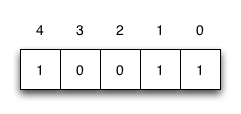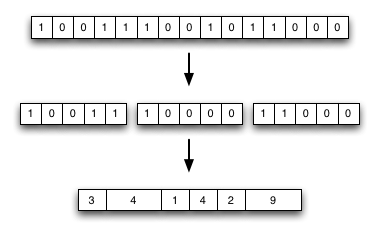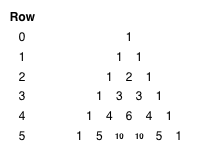Generating Binary Permutations in Popcount OrderI’ve been keeping an eye on the search terms that land people at my site, and although I get the occasional “alex bowe: fact or fiction” and “alex bowe bad ass phd student” queries (the frequency strangely increased when I mentioned this on Twitter) I also get some queries that relate to the actual content.

One query I received recently was “generating integers in popcount order”, I guess because I mentioned popcounts (the number of 1-bits in a binary string) in a previous post, but the post wasn’t able to answer that visitors question.

What would this be used for? Among other applications, I have used it for generating a table of numbers ordered by popcount, which I used in a compression algorithm: by breaking a bitstring into fixed-length chunks (of B bits) and replacing them with a (P, O) pair, where P is the block’s popcount which can be used to point to the table where each entry has the popcount P, and O is the offset in that subtable. Then P can be stored with log2(B + 1) bits – we need to represent all possible P values from 0 to B – and O can be stored with log2(binomial(B, P)) bits.Note that the bit-length of P varies; binomial represents the binomial coefficient, which can be seen in Pascal’s triangle expanded to row 5:So binomial(5, x) for x = 0, 1, … , 5 yields the sequence 1, 5, 10, 10, 5, 1 – some things take more bits than others. Once you know the P value, you will know how many bits the O value is, so you can read it that way. This means access is O(N) (since each values position relies on the previous P values), but you can build an index on top of that to allow O(1) lookup. But all this is a story for another time ;) .

Here is the code:

def next_perm(v):
"""
Generates next permutation with a given amount of set bits,
given the previous lexicographical value.
Taken from http://graphics.stanford.edu/~seander/bithacks.html
"""
t = (v | ( v - 1)) + 1
w = t | ((((t & -t) / (v & -v)) >> 1) - 1)
return w

This will take a number with a certain popcount, and generate the next number with the same popcount. For example, if you feed it 7, which is 111 in binary,
you will get 1011 back – or 11 – the next number with the same popcount (lexicographically speaking).

To find the first number of a given popcount, you can use this:

def element_0(c):
"""Generates first permutation with a given amount
of set bits, which is used to generate the rest."""
return (1 << c) - 1

I should clear up what lexicographically means in the context of numbers. Well, it’s actually the same as any other symbols (such as an alphabet), 0 is the symbol that comes before 1 (if it helps, you can picture 0 as a and 1 as b):

00111 aabbb
01011 ababb
01101 abbab
01110 abbba
10011 baabb
10101 babab
10110 babba
11001 bbaab
11010 bbaba
11100 bbbaa

Psuedocode

Looking at the above pattern, here is some loose pseudocode that may help us understand how the above bithacks work:

1. Set i to the position of the rightmost bit
2. Stop if there are no set bits, or if we have looked at all the bits (i >= length of bitstring)
3. If the i+1th bit (one place to the left) is 0: move the ith bit left
4. Otherwise, if the i+1th bit (one place to the left) is 1: set i to i + 1 and repeat from 2.
5. Shift the bits on the range [0, i] right so that the rightmost bit is in position 0

Explanation

Understanding element_0() is pretty easy. 1 << c is the same as moving a 1 to position c, then -1 sets all the bits from 0 to c – 1, giving c set bits:

c = 4
1 << c = 10000
10000 - 1 = 01111

next_perm() is a bit more complicated. The v | (v -1) in t = (v | (v - 1)) + 1 right-propagates the rightmost bit. Allow me to show an example: 01110 | 01101 = 01111

In the case of 0, this isn’t quite correct: 00000 | 11111 = 11111 But it’s okay because we proceed to add 1 to this value (which returns it to zero). This increment, combined with the right-propagation, will do step 2, 3, and part of step 5 above. For example, 10100 -> 10111 -> 11000.

We are on our way to generating integers by popcount in lexicographical order.

Now let’s break down the next line, w = t | ((((t & -t) / (v & -v)) >> 1) - 1). Bitwise equations of the form (x & -x) isolate the rightmost bit:
01110 & 10010 (two's complement) = 00010

If you take the two’s complement, you invert a numbers bits and then add 1. If you think about it, this means there are 0s where there were 1s, and 1s where there were 0s, and adding 1 bumps the rightmost 1 left and sets the subsequent right bits to 0. This means that the only position that will remain set in both numbers is the the rightmost 1-bit.

So let R(x) denote the isolated rightmost bit of x, then for x = 01110 we calculate t = 10000, R(x) = 00010 and R(t) = 10000.

Following the calculation of w, we need to divide them: R(t) / R(x) = 10000 / 00010 = 01000

Shift to the right by 1: 00100

Subtract 1: 00011

Then we bitwise-or them to stick them together: 10000 | 00011 = 10011. This corresponds to our table above :)

So w = t | ((((t & -t) / (v & -v)) >> 1) - 1) corresponds to the rest of step 5 (the moving part, t was the zeroing part of moving the sub-range) in our pseudocode. Well, kind of anyway, there are a few steps happening in parallel, but the pseudocode was only loosely explaining what was happening :)

Testing it out

def gen_blocks(p, b):
"""
Generates all blocks of a given popcount and blocksize
"""
v = initial = element_0(p)

while (v >= initial):
yield v
v = next_perm(v) & block_mask

>>> for x in gen_blocks(3, 5): print bin(x, 5)
...
00111
01011
01101
01110
10011
10101
10110
11001
11010
11100

Note: bin is just a function I found online for printing binay numbers and isn’t important to this post, but you can find it here if you need one.

Then of course you can loop through all values of P from 0 to B to build the complete table.

Questions? Comments? Flames? I wanna hear em :)

 – Check out Tables by Munro, 1996, and Succinct indexable dictionaries with applications to encoding k-ary trees and multisets by Raman et al, 2002 for a first step into this stuff. Also check out my honours thesis, 2010 for a recent look at succinct data structures. I will write a blog post about this stuff sooner or later :P